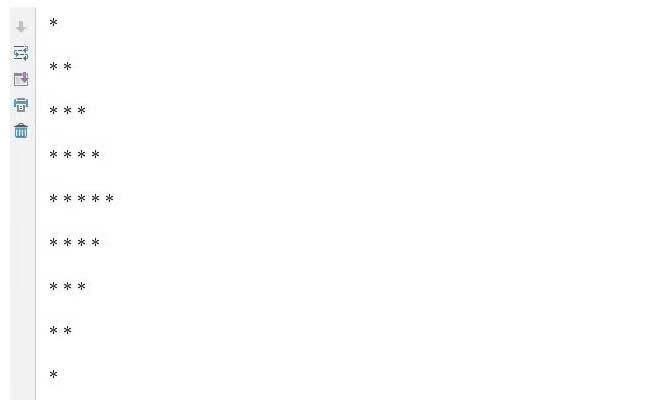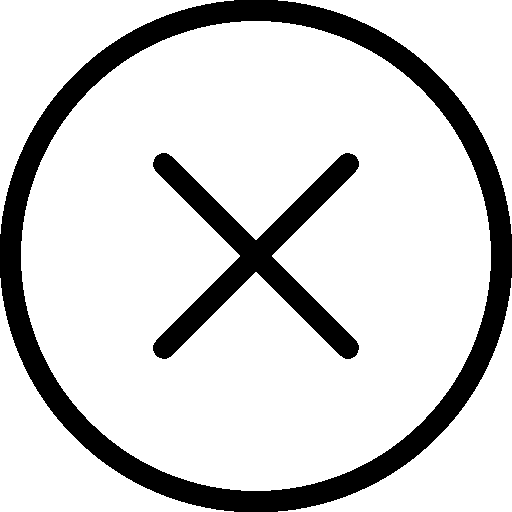• 写文章
• 提问

# 如何使用python3输出图形？```i=1
while i<=9: #整体九行*
j = 1
k = 9
while j <= i <= 5: #第一个while 组成上半部
print ('* ', end='')
j += 1 #j循环i次，代表这一行有i个*，如当i=5时，j循环5次，第五行5个*
while k >= i > 5: #第二个while 组成下半部
print ('* ', end='')
k -= 1 #k循环k-i+1次，如当i =7时，k就取7,8,9,循环3次，第七行3个*
print ('\n')
i += 1```